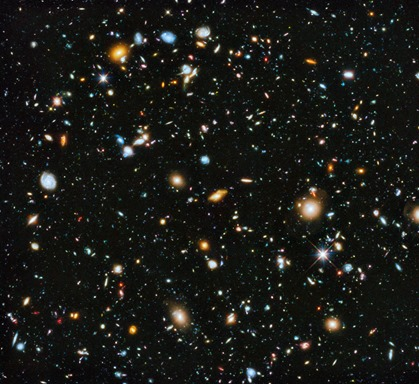## 4 May 2016

### 3D Universe ‘frozen in’ at the time of the Big BangThe question of why space is three dimensional (3D) and not some other number of dimensions has puzzled philosophers and scientists since ancient Greece. Space-time overall is four-dimensional, or (3+1)-dimensional, where time is the fourth dimension. It's well-known that the time dimension is related to the second law of thermodynamics: time has one direction (forward) because entropy (a measure of disorder) never decreases in a closed system such as the universe.

In a new paper published in EPL, researchers have proposed that the second law of thermodynamics may also explain why space is 3D.

"A number of researchers in the fields of science and philosophy have addressed the problem of the (3+1)-dimensional nature of space-time by justifying the suitable choice of its dimensionality in order to maintain life, stability and complexity," co-author Julian Gonzalez-Ayala, at the National Polytechnic Institute in Mexico and the University of Salamanca in Spain, told Phys.org.

"The greatest significance of our work is that we present a deduction based on a physical model of the universe dimensionality with a suitable and reasonable scenario of space-time. This is the first time that the number 'three' of the space dimensions arises as the optimization of a physical quantity."

The scientists propose that space is 3D because of a thermodynamic quantity called the Helmholtz free energy density. In a universe filled with radiation, this density can be thought of as a kind of pressure on all of space, which depends on the universe's temperature and its number of spatial dimensions.

Here the researchers showed that, as the universe began cooling from the moment after the big bang, the Helmholtz density reached its first maximum value at a very high temperature corresponding to when the universe was just a fraction of a second old, and when the number of spatial dimensions was approximately three.

The key idea is that 3D space was "frozen in" at this point when the Helmholtz density reached its first maximum value, prohibiting 3D space from transitioning to other dimensions.

This is because the second law allows transitions to higher dimensions only when the temperature is above this critical value, not below it. Since the universe is continuously cooling down, the current temperature is far below the critical temperature needed to transition from 3D space to a higher dimensional space. In this way, the researchers explain, spatial dimensions are loosely analogous to phases of matter, where transitioning to a different dimension resembles a phase transition such as melting ice—something that is possible only at high enough temperatures.

"In the cooling process of the early universe and after the first critical temperature, the entropy increment principle for closed systems could have forbidden certain changes of dimensionality," the researchers explained.

The proposal still leaves room for higher dimensions to have occurred in the first fraction of a second after the big bang when the universe was even hotter than it was at the critical temperature.

Extra dimensions are present in many cosmological models, most notably string theory. The new study could help explain why, in some of these models, the extra dimensions seem to have collapsed (or stayed the same size, which is very tiny), while the 3D space continued to grow into the entire observable universe.